# How to get Start Period of Time Range?Hi experts,

Is there a way to get start period of a time range in a LineItem?

Regards,

Inoue

Tagged:

•Use Period(start())  in your month formatted line item , time range dimension-ed module.

Similarly, you can check other functions like Currentperiodstart() and end() with respect to your requirement.

https://help.anaplan.com/anapedia/Content/Calculation_Functions/All/START.html

~Abhi

•Create a number formatted line item called Cumulate with the formula:

`CUMULATE(1)`

Then create a day/week/month formatted line item called First period with the formula

`IF Cumulate = 1 THEN ITEM(Time) ELSE PREVIOUS(First period)`

Set the summary of this line item to MIN if required

Result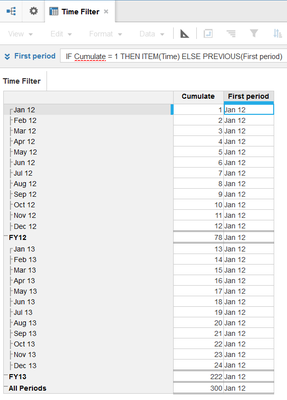Let me know if this works

Regards,

Anirudh

•Hi Abhi,

Just the START formula would not work. Because when it is dimensioned against month it will return that particular month itself instead of the beginning of the time range.....

Regards,
Anirudh
•The simplest way to achieve this is to create a time properties model set with year time period.

Create a single line item dimensioned by time period month

Use the following formula - Period(start())

This will generate the first time period of the year which you can then pull into your target modules.

The targets will need to be time dimensioned and will require the following formula

YEARVALUE(Time Properties Year.First Period)

This is the simplest way to reference first period for any given year.

•Period(start()) is the way to go as others have said, but also change the summary to First Non Blank.

First, make sure Total of All is turned on in your Time Range.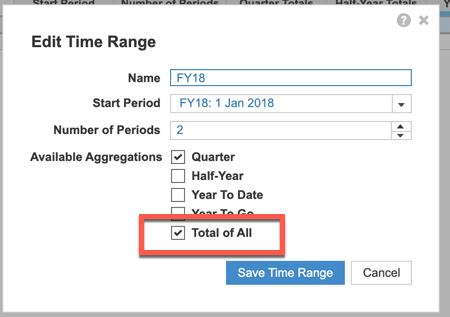Create a SYS Time Filter module using the Time Range, in my case FY18.  Create a line item with Period(start()) with a format of Time Period (month) if you are using month.  Next, change the summary to First non-blank.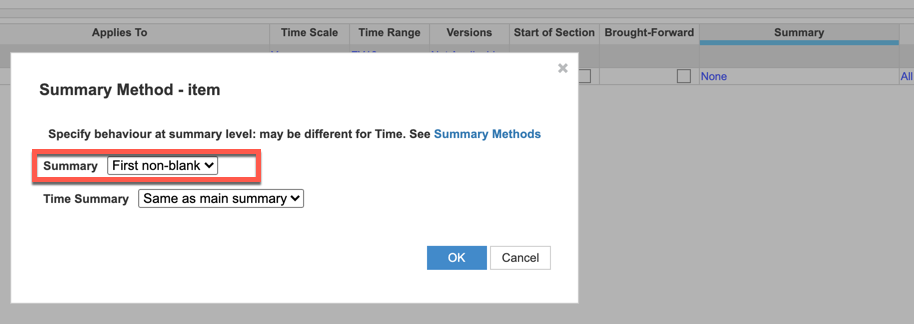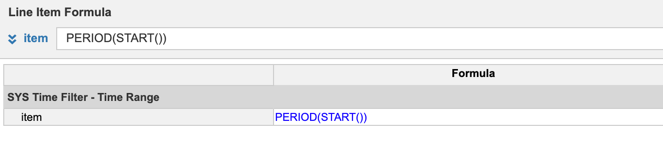for a result of: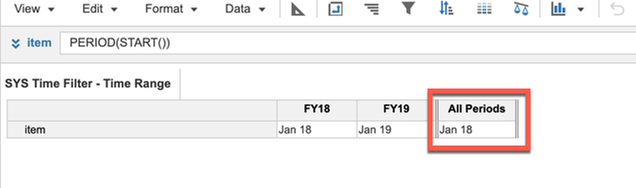Now, you can get the final solution in a line item using a Select statement which is ok because it is structural data.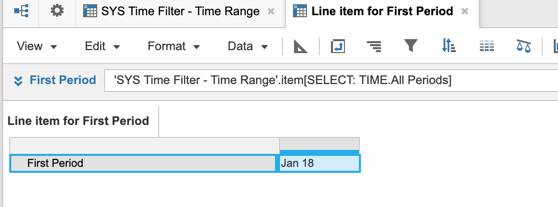Hope this helps,

Rob